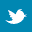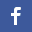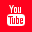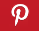Completely Solved C, C++ Programs Assignment.Quick Search Database Videos Tutorials Ebooks Forums FAQ Aboutus Household Industrial Manufacturing Service Shopping Transportation### C program to take a string and display the count of each character without repetition.

 Filed Under: C Assignments

Problem Statement:
Write a program to take a string and display the count of each character without repetition.

Theory:
The count of the occurrence of a particular character in a string is known as its frequency.In this program the characters are selected from the string one at a time and checked if its count has already been calculated previously else its frequency is determined after traversing through the entire length of the string and the result is stored in an array.

Algorithm:
Begin
Read a //Array to store inputted string
for i←0 to less than length of a,increment I by 1
Convert a[i] to upper case
Declare array b c of datatypes char and int respectively
for i←0 to less than 50,increment I by 1
b[i] ←\0
c[i] ←0
for i←0 to less than length of a,increment I by 1 //loop to traverse
set f ←0
for j ← 0 to less than equal to k,increment j by 1
if b[j] is equal to a[j]
f←1
increment c[j] by 1
break
if f is equal to 0
increment k by 1
b[k] ←a[i]
increment c[k] by 1
//Printing frequency
for i←1 to less than equal to k,increment I by 1
print frequency of b[i] is c[i]
End

Proglem Statement:
//program to print frequency of each character present in it without repetition//
#include<stdio.h>
#include<string.h>
void main()
{
char b;
int c;
int i,j,k=0,f;
char a;
printf(“Enter a string \n”);
gets(a);
for(i=0;i<strlen(a);i++);
a[i]=toupper(a[i]);
for(i=0;i<50;i++)
{
b[i]=’\0’;
c[i]=0;
}
for(i=0;i<strlen(a);i++)
{
f=0;
for(j=0;j<=k;j++)
{
if(b[j]==a[i])
{
f=1;
c[j]++;
break;
}
}
If(f==0)
{
++k;
b[k]=a[i];
c[k]++;
}
}
for(i=1;i<=k;i++)
printf(“Frequency of %c is %d \n”,b[i],c[i]);
}

Output:
Enter a string
There are 26 alphabets in the english language
Frequency of T is 3
Frequency of H is 4
Frequency of E is 7
Frequency of R is 2
Frequency of is 7
Frequency of A is 5
Frequency of 2 is 1
Frequency of 6 is 1
Frequency of L is 3
Frequency of P is 1
Frequency of B is 1
Frequency of S is 2
Frequency of I is 2
Frequency of N is 3
Frequency of G is 3
Frequency of U is 1

Discussions:
• The string should either be converted to the upper case or to the lower case to prevent printing frequency of a particular character in two different segments,one for the upper case and one for the lower case.
• The counter array should be initialized with 0 so as to prevent initialization of a garbage value and furthermore incrementing it,thus outputting wrong results.
• A flag variable should be incorporated so as to determine whether the occurrence of a particular character has already been determined earlier.Back to main directory:Software Practical